Ex 13.3

Chapter 13 Class 10 Surface Areas and Volumes (Term 2)
Serial order wise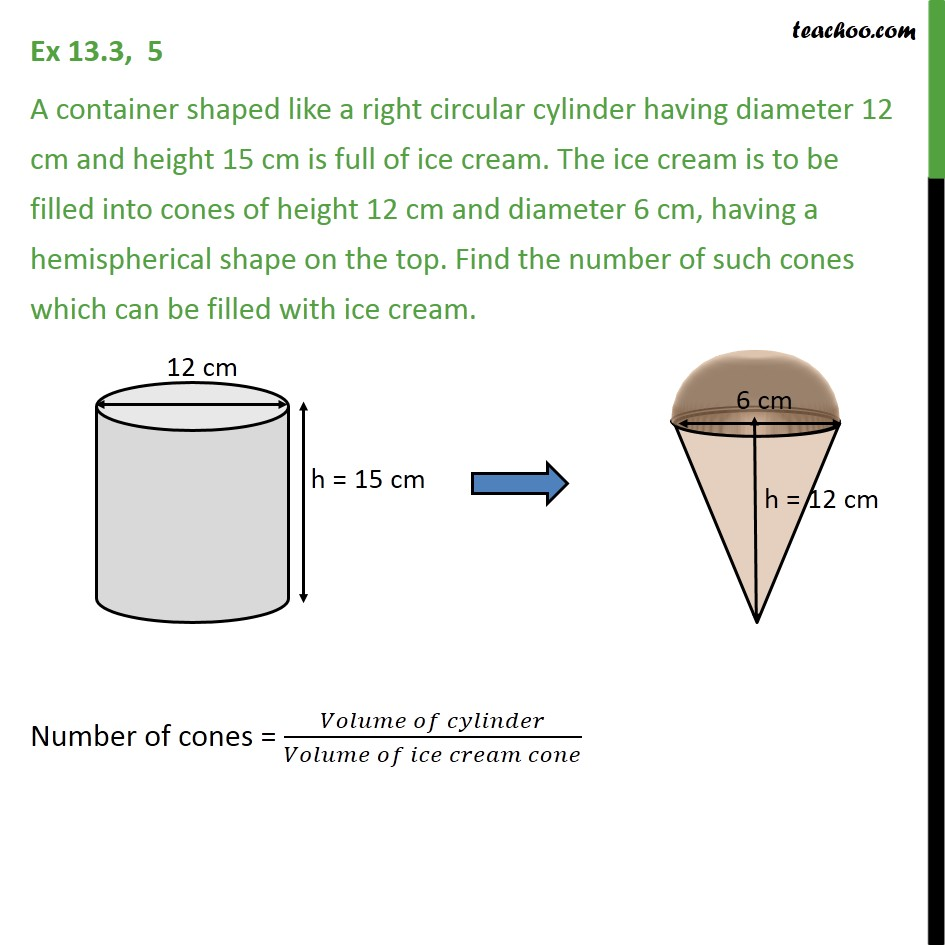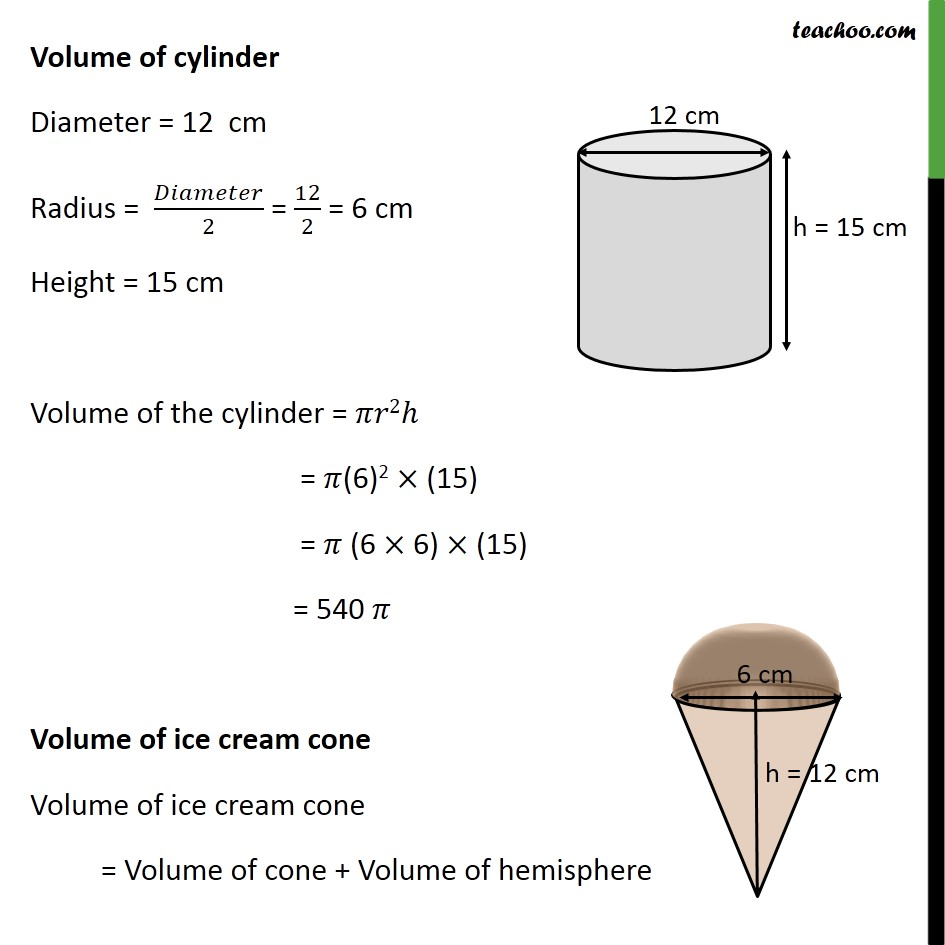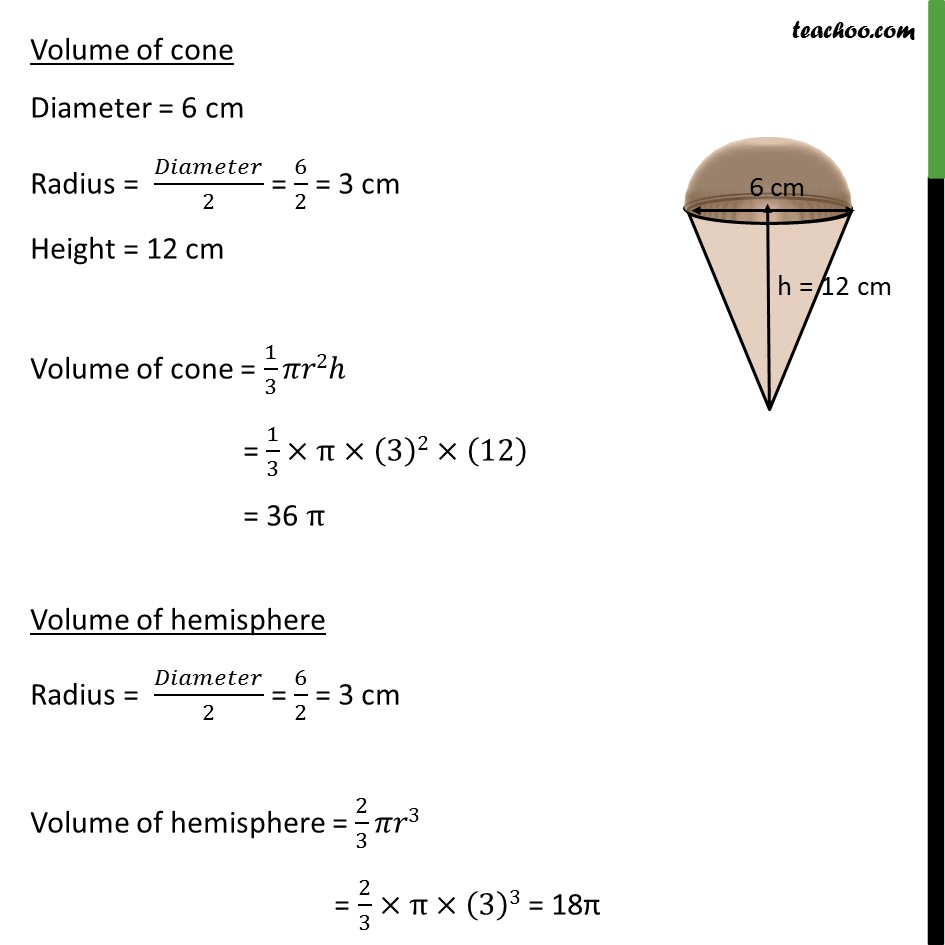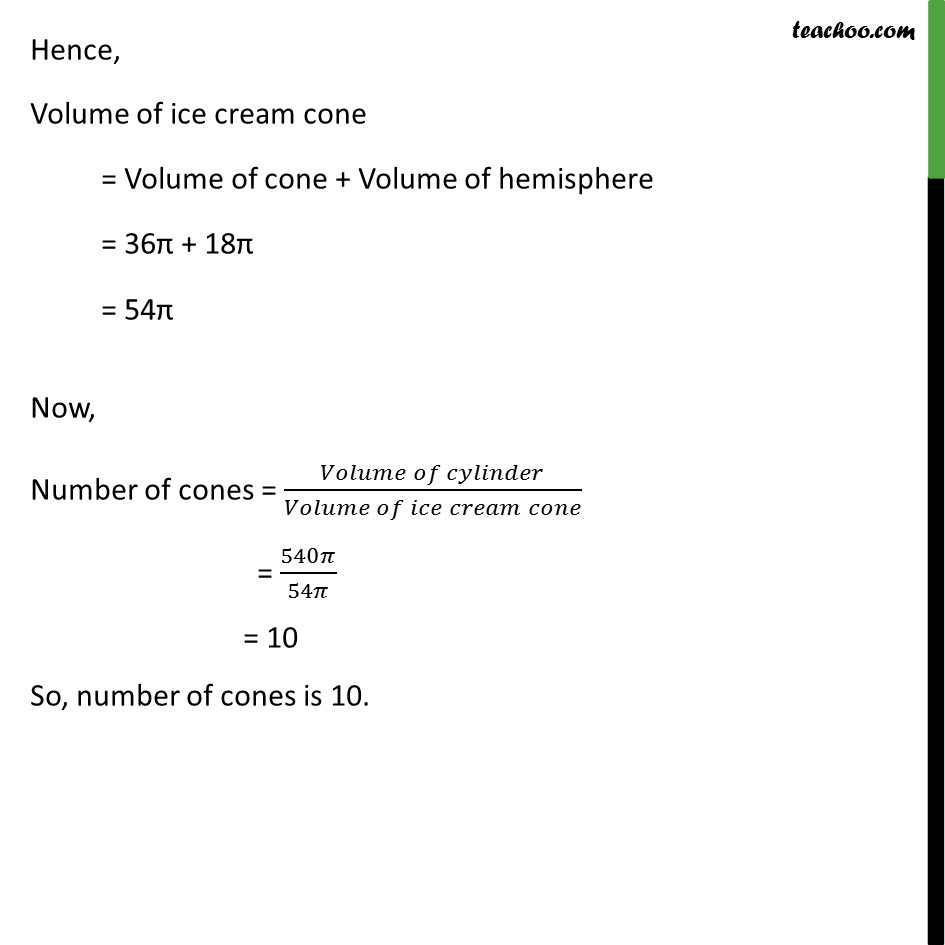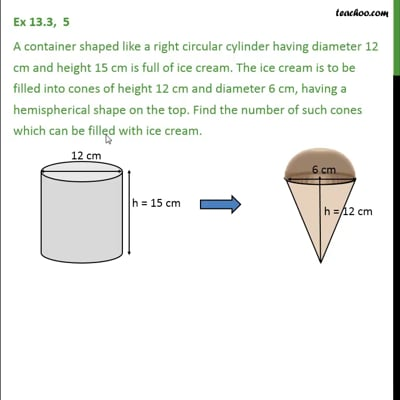This video is only available for Teachoo black users

### Transcript

Ex 13.3, 5 A container shaped like a right circular cylinder having diameter 12 cm and height 15 cm is full of ice cream. The ice cream is to be filled into cones of height 12 cm and diameter 6 cm, having a hemispherical shape on the top. Find the number of such cones which can be filled with ice cream. Number of cones = ( )/( ) Volume of cylinder Diameter = 12 cm Radius = /2 = 12/2 = 6 cm Height = 15 cm Volume of the cylinder = 2 = (6)2 (15) = (6 6) (15) = 540 Volume of ice cream cone Volume of ice cream cone = Volume of cone + Volume of hemisphere Volume of cone Diameter = 6 cm Radius = /2 = 6/2 = 3 cm Height = 12 cm Volume of cone = 1/3 2 = 1/3 (3)2 (12) = 36 Volume of hemisphere Radius = /2 = 6/2 = 3 cm Volume of hemisphere = 2/3 3 = 2/3 (3)3 = 18 Hence, Volume of ice cream cone = Volume of cone + Volume of hemisphere = 36 + 18 = 54 Now, Number of cones = ( )/( ) = 540 /54 = 10 So, number of cones is 10.